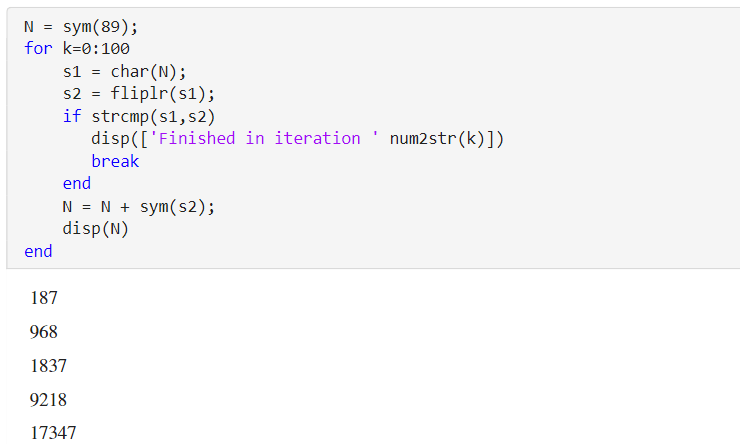# Handling Large Integers to Solve the 196 Problem

This example shows how to work with large integers and their decimal representation using the Symbolic Math Toolbox™.### Palindromes

A character string is called a palindrome if it is the same when read backwards. A positive integer is called a palindrome if its decimal representation is a palindrome. For example, 191, 313 and 5885 are all palindromes.

Consider the following algorithm

• Start with any positive integer $N$ and add it to its mirror image.

• Repeat this step with the resulting number until you obtain a palindrome.

For example, let N=89; then the first 3 iterations give ...

`$89+98=187$`

`$187+781=968$`

`$968+869=1837$`

eventually after 24 iterations you would arrive at the palindrome 8813200023188.

```N = sym(89); for k=0:100 s1 = char(N); s2 = fliplr(s1); if strcmp(s1, s2) disp(['Finished in iteration ' num2str(k)]) break end N = N + sym(s2); disp(N) end```
`$187$`
`$968$`
`$1837$`
`$9218$`
`$17347$`
`$91718$`
`$173437$`
`$907808$`
`$1716517$`
`$8872688$`
`$17735476$`
`$85189247$`
`$159487405$`
`$664272356$`
`$1317544822$`
`$3602001953$`
`$7193004016$`
`$13297007933$`
`$47267087164$`
`$93445163438$`
`$176881317877$`
`$955594506548$`
`$1801200002107$`
`$8813200023188$`
```Finished in iteration 24 ```

### The 196-Problem

Does the algorithm terminate for every $N$?

The problem is still open, and palindrome aficionados have invested many CPU years into the $N=196$ case which gave the problem its name. In order to play with this problem in MATLAB®, symbolic integers are useful because their size is unlimited. Use the function `sym` to convert strings of decimal digits to symbolic integers, and `char` (not `num2str` !) to convert back.

Investigating the famous $N=196$ case produces truly huge numbers. To see how many decimal digits an integer has, simply use `log10` :

```N = sym(196); for k=0:1000 s1 = char(N); s2 = fliplr(s1); N = N + sym(s2); end disp(['Number of digits after ' num2str(k) ' iterations: ' char(ceil(log10(N)))]);```
```Number of digits after 1000 iterations: 411 ```